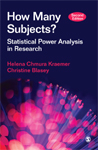# How Many Subjects?: Statistical Power Analysis in Research

This book is a simple introduction for nonstatisticians to power analysis and sample size determination. It clearly illustrates why sample sizes need to be sufficiently large, so that the experiment has good power properties and hence low type II error rates. The authors introduce a simple technique of statistical power analysis that allows researchers to compute approximate sample sizes and power for a wide variety of research designs. Because the same technique is used with only slight modifications for different statistical tests, researchers can easily compare the sample sizes required by different designs and tests to make cost-effective decisions in planning a study. These comparisons, emphasized throughout the book, demonstrate some important principles of design, measurement, and analysis that are not given sufficient emphasis in other texts.

•••